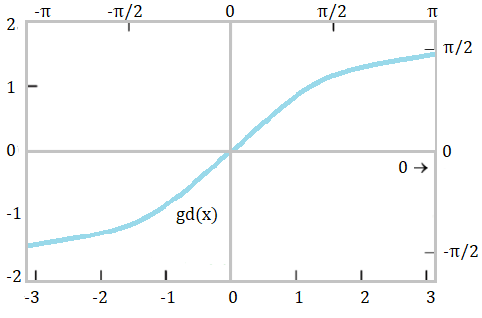# Gudermannian Function (Gudermann)The Guderman function, Θ = gd(x) is an odd function, named after the 19th century German mathematician Christopher Gudermann. It is also called the Gudermannian function, or just “the Gudermannian”.

This function is particularly useful when dealing with circular functions and hyperbolic functions, because it negates the need to use imaginary arguments (Fogiel, 1984). The Gudermannian is included in various applied mathematical definitions, including the angle of parallelism in hyperbolic geometry (½π = gd x) and the transverse Mercator projection, which uses a horizontal cylinder oriented tangent to a Meridian.

## Gudermann Function Definition

This function can be defined in various ways. One of the most common (and simplest) is:

gd x = 2 tan-1 ex – π/2

## Alternative Definitions

One definition is as the indefinite integral of the hyperbolic secant (Spanier & Oldham, 1987):It can also be defined as:Various other definitions can be found in the literature, including:

• gd x = arcsin(tan x) = arctan (sinh x) = arccsc(coth x)
• gd x = 2 arctan [tanh(½x)]
• gd x = 2 arctan(ex – ½π
• gd x = sgn (x) · arccos(sech x) = sgn(x) · arcsec(cosh x)

Where:

## References

Beyer, W. H. “Gudermannian Function.” CRC Standard Mathematical Tables, 28th ed. Boca Raton, FL: CRC Press, p. 164, 1987.
Ogden, J. & Fogiel, M. (Eds.). (1984). Handbook of Mathematical, Scientific, and Engineering Formulas, Tables, Functions, Graphs, Transforms. Research and Education Association.
Spanier, J. & Oldham, K. (1987) An Atlas of Functions. Taylor & Francis.
Zwillinger, D. (Ed.). “Gudermannian Function.” §6.9 in CRC Standard Mathematical Tables and Formulae, 31st ed. Boca Raton, FL: CRC Press, pp. 530-532, 2002.

CITE THIS AS:
Stephanie Glen. "Gudermannian Function (Gudermann)" From StatisticsHowTo.com: Elementary Statistics for the rest of us! https://www.statisticshowto.com/gudermannian-function-gudermann/